Register Journal

Visitors Counter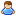Today
2213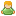Yesterday
1982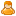This week
2213Last week
13811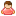This month
27345Last month
58345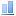All days
2979822

Malaya Journal of Matematik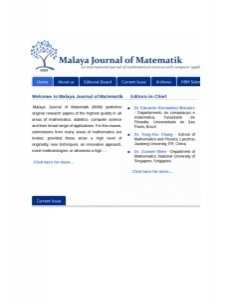• Year publication
• 2012
• Frequency
• 4
• Article Publishing Frequency
• 0
• CGIJ OAJI
• 0.000
• Abbreviation
• Country
• India
• ISSN (print)
• 2319-3786
• ISSN (online)
• 2321-5666
• Editor in Chief
• Dr. Eduardo Hernandez Morales
• ISI
• All articles
• 0
• Date added to OAJI
• 24 Oct 2014
• Scopus
• All issues
• 0
• Free access
• DOAJ
• Full text language
• English
• Journal discipline
• Journals
• Physico-mathematical Sciences
• 52
• Journal description
• Malaya Journal of Matematik (MJM) publishes original research papers of the highest quality in all areas of mathematics, statistics, computer science and their broad range of applications. For this reason, submissions from many areas of mathematics are invited, provided these show a high level of originality, new techniques, an innovative approach, novel methodologies, or otherwise a high level of depth and sophistication. Any work that does not conform to these standards will be rejected. Areas covered include (but are not limited to) algebra, algebraic topology, advanced calculus, advanced numerical methods, artificial neural networks, calculus and trigonometry, complex analysis, computational fluid dynamics, control theory, differential topology, differential geometry, dynamical systems, chaos and fractals, fluid dynamics and applications, fractional differential equations, functional analysis, fuzzy logic, general topology, genetic algorithms, linear algebra, linear programming models, mathematical modeling, markov chains and applications, modern algebra, nonlinear analysis, nonlinear programming models, number theory, numerical analysis, operations research, optimization theory , ordinary differential equations, partial differential equations, probability and queuing theory, random processes, real analysis, singular perturbation theory, stochastic differential equations, stochastic modeling, univalent functions. This journal also aims to cover the algorithmic and computational aspects of these disciplines. Hence, all mathematics, statistics and computer science contributions of appropriate depth and relevance to the above mentioned applications in mathematical sciences are welcome. More detailed indication of the journal’s scope is given by the subject interests of the members of the board of editors. All papers will undergo a thorough peer reviewing process unless the subject matter of the paper does not fit the journal; in this case, the author will be informed promptly. Every effort will be made to secure a decision in three months and to publish accepted papers within one month. MJM publishes four volumes annually and each volume comprises of one issues appearing in January, April, July, and October and is a joint publication of the University Press.
• Journal is indexed by